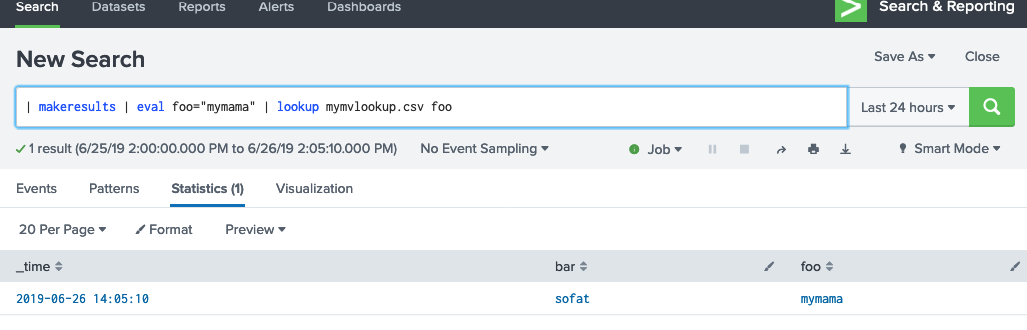# Splunk Multi-value csv lookups

This is a `TIL` post. Thanks to Matt, Duane, and Dave H for this.

Turns out there is a hidden option (supported, but not in docs) for Splunk’s `outputlookup` command.

Namely, the option `output_format` which has the following values:

• `splunk_sv_csv` (default)
• `splunk_mv_csv`

What does this do? This controls how Splunk will output fields in a lookup, and allow you to output fields in a correct multi-value fashion.

Here’s an example. Let’s say we have a field where we might have a different values, but we want to have one entry from a `lookup` command. Without a properly formatted multi-value aware lookup file, you would have to have two entries. Let’s look at some searches.

## Normal CSV lookup

To create a sample lookup, we can use:

``````| makeresults | eval foo=mvappend("yomama","mymama"), bar="sofat" | fields - _time| outputlookup mylookup.csv
``````

The resulting `mylookup.csv` looks like this:

``````bar,foo
sofat,"yomama
mymama"
``````

Notice the newline in the results.

If we try to perform a lookup against it, won’t work:

``````| makeresults | eval foo="mymama" | lookup mylookup.csv foo
``````

This results in an empty `bar` field.

## MV CSV lookup

If we create a mv lookup:

``````| makeresults | eval foo=mvappend("yomama","mymama"), bar="sofat" | fields - _time| outputlookup mymvlookup.csv output_format=splunk_mv_csv
``````

The resulting CSV looks like this:

``````bar,foo,"__mv_bar","__mv_foo"
sofat,"yomama
mymama",,"\$yomama\$;\$mymama\$"
``````

So, lets use that in a lookup:

``````| makeresults | eval foo="mymama" | lookup mymvlookup.csv foo
``````

Bringo. We get some results: